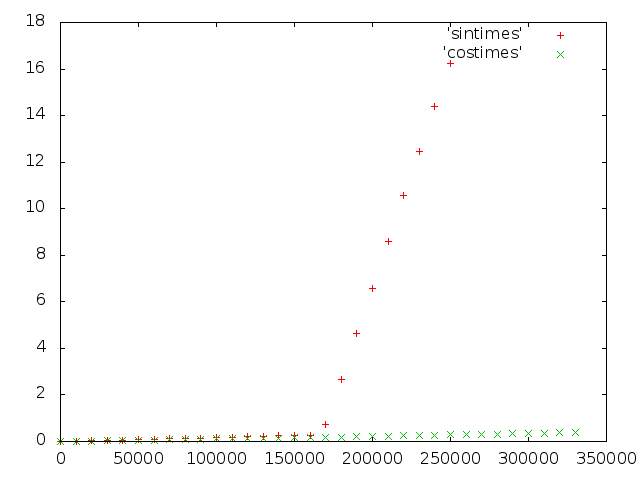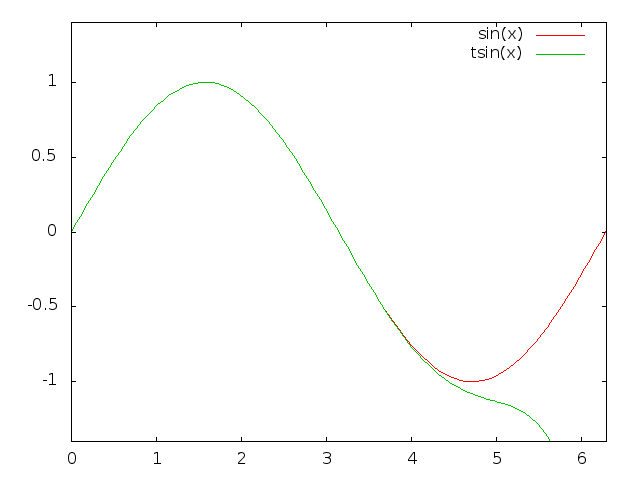## Thursday, October 3, 2013

### Help, my sin() is slow, and my FPU is inaccurate!

Recently, I started writing code to do a simulation of biological system, and I got stuck after 20 lines. My test code tried to go through a million time steps doing basically nothing, and it was taking a minute to do it. However, if I reduced this to only 100,000 time steps, it zoomed along.Number of steps versus runtime for sin() and cos()

So I checked the usual things - was there a CPU frequency scaling problem, was my CPU overheating, etc, but none of that was the case. Then I changed one call to sin() by a call to cos().. and everything was fast again.

After some further testing, I also discovered that changing M_PI to 3.141 also made things fast again. Color me confused!

So, I delved into the assembly output, where I found that nothing really changed in my code when I changed M_PI or sin() to cos(). What I did note was that an actual call was being made to a sin() function in the C library.

Trawling the web found various bug reports about sin() and cos() being both inaccurate and slow at times. And this blew my mind. I first got access to a computer with a floating point unit in the late 1980s. And over the past thirty years, I’ve been assuming (correctly) that an FPU would come with a dedicated SINCOS instruction. I had also assumed (incorrectly!) that since it came with the CPU, it would be correct & fast, and people would be using it!

It so turns out that what the FPU offers is neither correct, nor fast. And in fact, it appears nobody is even using the FPU trigonometry instructions. So what gives?

## Range reduction

Range reduction is what gives. It is entirely possible to very rapidly calculate sin(x) for small x using a simple approximation formula, for example, a Taylor series. Tables can also be used, and for hardware there is a suitable algorithm known as CORDIC (for COordinate Rotation DIgital Computer).

Such approximations only work well for small input though, but since sin() and cos() are periodic, it is entirely possible to map a large input angle to one that is small again.Actual sin(x) versus 11th order Taylor series, tsin(x)

So let’s say we are trying to calculate sin(1 + 600π), we can map this to trying to calculate sin(1) - the answer will be identical.

To do so, a computer program must take the input value offered, and remove sufficient even multiples of π that the resulting value is small enough that our approximation is accurate.

And here’s the rub. If our input can be all values attainable by a double precision floating point number, to perform this range reduction naively requires knowing π to an astounding precision, a precision unattainable itself in floating point hardware!

It is easy to see how this works - even a tiny error in the value of π adds up, in our example of sin(1 + 600π), an error of 1/1000 would still lead us to be off by 0.6!

Of course a CPU can do better than a 1/1000 error, but it will still never be able to reduce (say) 1022 back to the correct value. To see this in practice, the proper value of sin(9*1018) is -0.48200764357448050434, but if we ask my Intel FPU, it returns -0.47182360331267464426 which is off by more than 2%. To its credit, if we ask the FPU to calculate the sine of larger numbers, it refuses.

So.. this explains why a programming language can’t rely on the FPU to calculate proper values. But it still does not explain how a higher level language might solve this.

## Way back to 1982

The problem of range reduction was known to be hard - so hard that programmers were urged just not to try to calculate the sine of very large angles! “You do the range reduction”. This however is weak sauce, and makes life unnecessarily hard. For example, if modelling the response of a system to a sinusoid input of f Hz, it is typical to calculate F(x) = sin(2π * f * t) for prolonged periods of time. It would truly be inconvenient if we could only run simulations with well chosen time steps or frequencies so that we would be able to trivially adjust our ranges back to single digits.

And then, after telling programmers for years to clean up their act, suddenly two independent groups achieved a breakthrough. Mary H. Payne and Robert N. Hanek of Digital Equipment Corporation published the seminal paper “Radian Reduction for Trigonometric Functions”, paywalled to this day. Meanwhile Bob Corbett at UC Berkeley did not write a paper, but did independently implement the same technique in BSD.

I’d love to explain how it works, and it pains me that I can’t. It is very clever though! It is clear that we have to be grateful for Payne, Hanek and Corbett for inventing “infinite precision range reduction”! It appears to be in universal use, and it is quite astounding to realize that prior to 1982, it was effectively very hard to do trigonometry on very large numbers on the hardware that was available!

## So why was my original code so slow?

We now know that computers use actual code to calculate sin(x), and don’t leave it to a dedicated FPU instruction. But why was it fast up to 170,000 steps? It turns out that the sin(x) found in my C library contains various strategies for calculating the right answer, and it does indeed use the Payne-Hanek-Corbett technique, as implemented by IBM in their Accurate Mathematical Library.

If it all works out, some very rapid code is used. But if the library detects a case in which it can’t guarantee the required precision, it reverts to a functions actually called ‘slow()’. And they live up to the name. This is supposed to be rare though, so why was it happening to me?

Viz the test code:

int main(int argc, char **argv)
{
double t;
double stepsizeMS=0.1;
double Hz=1000;
double val=0;
unsigned int limit = argc > 1 ? atoi(argv) : 200000;

for(unsigned int steps = 0; steps < limit; ++steps) {
t = steps * stepsizeMS;
val = sin(2.0*M_PI*Hz*t);
printf("%f %f\n", t, val);
}
}
See if you can spot it. I didn’t, but the most excellent Nicolas Miell did. This code is effectively calculating the sine of exact multiples of π since Hz*stepsizeMS equals 100.0000, and steps is an integer. And apparently, after around 100,000 steps, this triggers the glibc libm implementation to revert to one of the ‘slow()’ functions.

This also explained why the problem went away by changing sin() into cos(), or my removing only a tiny bit of accuracy from M_PI - we no longer hit the slow path all the time.

Now, I’m not that happy that the system C library exhibits such slowdowns (which have been noted by others, see reference 2), but in this case my simulation would be useless in any case - I’d only be exerting a force of 0 anyhow, and never achieve anything!

It does turn out there are libraries that offer superior performance, like:

## Summarizing

Calculating (co)sines is done “manually”, and may proceed at different speeds depending on your input. The output of your FPU might be wrong, and many FPUs refuse to process really large inputs. Better libraries than your standard system library might be needed if you truly need high performance.

## References

1. Argument Reduction for Huge Arguments: Good to the Last Bit by K. C. Ng and the members of the FP group of SunPro - http://www.validlab.com/arg.txt
2. The state of glibc libm (dire) - http://gcc.gnu.org/ml/gcc/2012-02/msg00469.html
3. Intel overstates FPU accuracy - http://notabs.org/fpuaccuracy/
4. A new range reduction algorithm - http://www.imada.sdu.dk/~kornerup/papers/RR2.pdf